# Comparison of the Link Budget with Experimental Performance of a WiMAX System

## Abstract

The performance of different modulation schemes of a broadband fixed wireless 802.16 (WiMAX) system in a sector is experimentally determined and compared to link budget calculations using different path loss models. A link budget based on path loss measurements of the actual WiMAX signal at 3.5 GHz for a typical residential scenario in a suburban environment is determined. The link budget calculations show a reasonable agreement with actual performance measurements with certified WiMAX modems. Carefulness is advised when these models are used for the actual deployment of a WiMAX network in a specific area.

## 1. Introduction

Fixed Wireless Access (FWA) systems such as Worldwide Interoperability for Microwave Access (WiMAX), based on the standards IEEE 802.16  and HiperMAN , are being installed for field trials nowadays and become increasingly important. The advantages of fixed broadband wireless access over wired systems are mainly the lower cost and the flexibility for the deployment of the system. The competitiveness of WiMAX will largely depend upon the actual data rates and ranges that can be achieved.

In , link budget calculations (which are in fact coverage estimations) have been performed using different path loss models. However, these coverage estimations have not been validated by performance tests. Theoretical analyses and simulations of the performance of 802.16-based systems have been investigated in [3, 4, 612]. In , measurements of the carrier-to-interference-noise ratio (CINR) are shown and the actual measured performance of an 802.16-based system is investigated in .

The objective of this paper is to validate link budget calculations with actual performance results. Therefore the coverage is investigated in a sector for an IEEE 802.16-2004 system operating in Non-Line-of-Sight (NLOS) conditions at 3.5 GHz with a channel bandwidth of 3.5 MHz in a suburban environment of Ghent, Belgium. For this analysis a path loss model at 3.5 GHz is developed for suburban environments in Belgium for a transmitter and receiver height of 15 m and 2.5 m, respectively. Moreover, link budget calculations have been made using the path loss model and exisiting ones and compared to actual performance measurements of the WiMAX system.

Sections 2 and 3 describe the configuration and methodology of this analysis. The path loss model and link budgets are discussed in Section 4. The validation with actual performance measurements is described in Section 5. Finally, conclusions are presented in Section 6.

## 2. Configuration

### 2.1. Frequency Band Selection

When planning a FWA network, the operator has to make a choice between the available frequency bands. The selection of the frequency band to be used has a major effect on the dimensioning and planning of the FWA network.

Here, the 3.5 GHz FWA band was chosen. The decision was based on the fact that the band is licensed and interference is under control. Higher transmission powers are also allowed and the frequencies of the band are sufficiently low to obtain a better range and coverage than, for example, at 5.8 GHz. The 3.5 GHz FWA band is a licensed band and powers of 35 dBm into the BS antenna are allowed , while, for example, in the 2.4 GHz industrial, scientific and medical (ISM) band only an equivalent isotropically radiated power (EIRP) of 20 dBm is allowed.

### 2.2. Selected Parameters and Scenario

We will investigate an outdoor scenario for residential applications in Ghent. The height of the base station (BS)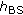is 15 m. We analyze the outdoor receiver (Rx) height of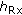equal to 2.5 m (representing the first floor of a house). At this height, the Rx will be typically installed in residential neighborhoods. The Rx is positioned at 2.5 m using a telecopic mast. The input power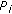to the BS antenna is 35 dBm (3.2 W).

The considered WiMAX system is based on the IEEE 802.16-2004 standard. The base station (BS) antenna is a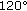sector antenna, with vertical polarization. The electrical beam tilt is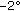(i.e.,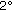below the horizontal plane). No mechanical tilt is used. The dimensions of the antenna are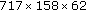mm. The Customer Premises Equipment (CPE) antenna is a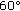directional antenna with dimensions of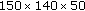mm. We consider outdoor CPE here. The frequency under consideration is 3520 MHz (downlink (DL) frequency). Figure 1(a) shows the BS on the roof and part of the environment, and Figure 1(b) shows the CPE antennas at a height of 2.5 m.

Table 1 summarizes the selected parameters and the scenario under investigation. The gains of BS and Rx correspond to a typical residential modem scenario, that is, a BS antenna gain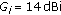and an Rx antenna gain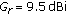. The BS feeder loss is 0.5 dB and the Rx feeder loss is 2.5 dB. The channel bandwidth (BW) of WiMAX systems can be varied from 1.25 to 28 MHz [1, 2]. In this paper we assume a BW of 3.5 MHz, because this bandwidth is typical for Europe and this BW was granted by the authority for the WiMAX field trial in Ghent.

The minimum required Rx sensitivity ([dBW]) can be calculated from the minimal required receiver noise input power (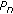[dBW]) and SNR (signal-to-noise ratio) by using the following formulas: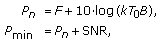(1)

where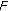is the receiver noise figure (6 dB),is Boltzmann's constant (1.38.10–23 Ws/K),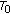the absolute temperature (290 K), andthe receiver noise bandwidth [Hz]. Table 2 shows the receiver sensitivity and SNR for the subscriber terminal of the residential modem system under consideration for a 3.5 MHz channel. Table 2 shows that the Rx sensitivity and required SNR depend on the type of modulation and coding rate. These values are valid for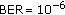(bit error rate) in a 3.5 MHz channel at 3.5 GHz.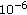Table 2 Rx sensitivity for the subscriber terminal of the WiMAX system for 3.5 MHz channel width (BER = , AWGN channel).

## 3. Methodology

Figure 2 shows a flow graph with the procedure to compare the link budget calculations with the actual performance of the considered system. First, the path loss and link budget methodology, which is used for estimating the ranges for the different modulation schemes of the WiMAX system will be explained. Secondly, the validation methodology, where the actual WiMAX performance measurements are executed, will be discussed.

### 3.1. Path Loss and Link Budget Methodology (Figure 2)

#### 3.1.1. Path Loss

The Rx antenna for the path loss measurements is an omnidirectional Jaybeam antenna type MA431X21 . For the adjustment of the height of the Rx we use a telescopic mast. The measurements are performed with a Rohde & Schwarz FSEM30 spectrum analyzer (SA). The output of the SA is sampled and stored on a laptop. The center frequency is 3520 MHz (DL), the frequency span is 20 MHz, and the resolution bandwidth (RBW) is 5 MHz. Figure 3 shows a trace measured with the SA (with described settings) of the actual WiMAX signal as a function of the frequency. The measurement positions are acquired with a GPS device. Using a car, the Rx is moved in the environment with a constant speed of 0.8 m/s.

The noise floor is determined for each measurement track. The noise floor for the SA-settings used here is about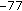dBm. Samples which are below the noise floor plus 10 dB (additional margin) are discarded. The measurement value will also depend on the detector mode: the root-mean-square (RMS) mode is used, as proposed in . To remove the fluctuations of the fast fading, the received signal strength is averaged and sampled according to the Lee criterion , that is, 50 samples for each 40 wavelengths. For an Rx height of 2.5 m about 60,000 samples are stored for averaging and further data processing. We consider distances from 30 m to 1300 m from the BS, because no connection could be obtained (see Sections 4 and 5) at further distances.

The calculation and tabulation of signal powers, gains, losses, and SNR for a complete communication link is called a link budget, which is a useful approach for the basic design of a communication system (Figure 2). To determine the link budget and the coverage range of the WiMAX system we use the parameters of Table 1 and the path loss models described in Section 4.1 (method of Section 3.1.1) and in . We have to remark that the link budget is calculated for the CPE antennas and not for the Jaybeam antenna, which is only used to determine the PL.

### 3.2. Validation Methodology (Figure 2)

The WiMAX system includes besides the base station (BS), also CPE (Customer Premises Equipment, also called WiMAX modems) for residential users. The WiMAX system supports BPSK, QPSK, 16-QAM, and 64-QAM modulations and provides a per-allocation adaptive modulation and coding scheme. This means that the WiMAX system should ensure the most robust link conditions with the highest data rate by optimally selecting the best physical mode in DL at each different location. Different CPEs are positioned in a minivan with the antennas mounted outside the car on a telescopic mast at a height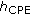of 2.5 m. For these outdoor measurements used for validation purposes (Figure 2), the CPE antennas have always been oriented in the direction where the Received Signal Strength Indication (RSSI) reaches a maximum. The direction of maximal RSSI is determined as follows. The CPE antenna is slowly manually rotated and at the same time the RSSI value is automatically displayed. By repeating this procedure a few times, the direction with maximal RSSI is obtained.

To obtain information of the several Radio Frequency (RF) characteristics such as modulation scheme, CINR, and received signal power, the webinterface of the CPE is used. These parameters can be retrieved with a simple tool, which stores the data in a text file. Only the DL is considered here.

The measurements (noted as "performance measurements'' here, not to be confused with the path loss measurements in Section 3.1) are performed at different locations in Ghent. Only that area in Ghent that is radiated by the main lobe of the base station antenna is considered. This area is divided into multiple zones separated 100 m from each other. We consider only zones at distances between 100 m and 1300 m from the BS. The first zone is the area within a distance of at least 100 m and at most 200 m from the BS, the second zone is the area within a distance of at least 200 m and at most 300 m from the BS, and so forth. For each zone three locations are arbitrarily selected, so in total 36 locations are analyzed (of which some of them did not acquire connection). At every location the RF parameters (particularly the assigned modulation scheme in DL) are retrieved each second for a period of 720 seconds (twelve minutes) by using the we tool. This measurement period is necessary to execute performance tests to obtain statistical relevant results. In Section 5 the results of these actual performance measurements will be compared to link budget calculations (presented in Section 4).

## 4. Path Loss Results

### 4.1. Path Loss Models

Path loss (PL) between a pair of antennas is the ratio of the transmitted power to the received power. It includes all of the possible elements of loss associated with interaction between the propagating wave and any objects between the transmitting and receiving antennas . The path loss is modelled according to a lognormal shadowing model. In this paper the median path loss is modelled as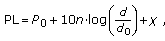(2)

whereis the distance in m,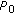is the path loss in dB at an arbitrarily chosen reference distance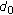in m, andis the dimensionless path-loss exponent.is chosen to be 100 m here (within the range of the measurements namely 30 m up to 1300 m). Furthermore,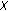is the shadowing fading variation and has a standard deviation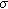. The interceptcan be determined in two ways:

(i)nonfixed intercept: the interceptis considered as a separate variable of which the value is determined through a least-squares fit,

(ii)fixed intercept: the interceptis chosen fixed and equal to the free-space path loss at reference distance, that is,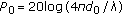, whereis the wavelength in m (method of ).

The one-slope model's parameters are determined for both nonfixed and fixed intercept. A comparison between nonfixed and fixed intercept path loss models is made to examine which approach provides a better fit to experimental data.

First, a fit with two parameters (and, model 1, i.e., nonfixed intercept) is executed. Figure 4 shows the PL at= 2.5 m as a function of the distance BS-Rx. The RMS deviation of the points of the figure is minimized with a linear regression fit, whereandare adjusted. We obtain for model 1 a path loss exponent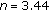and a standard deviation of 7.13 dB. The parameterequals 96.9 dB.

Secondly, we perform a fit where only the parameterof (2) is adjusted (model 2, i.e., fixed intercept) andis defined as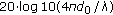, whereis the wavelength in m. Withm,is 83.38 dB. As a result, we obtain for model 2 a path loss exponent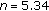and a standard deviation of 8.33 dB. Figure 4 shows the PL models at= 2.5 m as a function of the distance BS-Rx.

For model 1, the lowest standard deviation is obtained, indicating a better resemblance with the experimental data. This is of course due to the use of two parameters for the fit. The path loss exponent derived in  (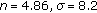) for the suburban environment (Erceg C model, single parameter ) is lower than the value obtained with model 2. This could be explained by the fact that the houses in the environments of  contain less brick and reinforced concrete material than the houses in this paper and that suburban streets of  are mostly wider than the streets in this paper. Suburban environments in Europe (such as cities like Ghent) are thus more dense than in, for example, the USA. The standard deviation determined in  for suburban environment is about the same as the value obtained with model 2.

### 4.2. Cumulative Distribution Function

To investigate the correctness of the models, the cumulative distribution function (CDF), that is,of the difference between the experimental data and the mean path loss model is analyzed. This CDF is then being fit using a CDF of a lognormal distribution (in dB). The RMS deviation is minimized with a linear regression fit, where the mean value is set to zero and the standard deviationis adjusted.

In Figure 5, the experimental CDF and the lognormal fit of the difference of the received powerand the received power according to the model are shown for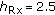m. The standard deviationof the lognormal fit equals 7.08 dB, which is close to the 7.13 dB of the experimental data.

Also the CDF of the difference between the logarithmic experimental data and the values predicted by model 2 is made and this CDF is being fit to a CDF of a lognormal distribution with mean value zero. The resulting standard deviationof the lognormal fit equals 8.71 dB (only a deviation of 0.38 dB from the experimental value ofof 8.33 dB). The experimental CDF and the lognormal CDF are also shown in Figure 5.

Both models deliver accurate results, but the use of the first model (nonfixed intercept) is preferred because of the following reason. In the literature it is generally accepted that shadow fading samples are lognormally distributed. The K-S (Kolmogorov-Smirnov) test is a goodness-of-fit test that can be performed on the shadow fading samples to ascertain this lognormality. The nonfixed intercept one-slope model passed the K-S test at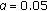level of significance; whereas the fixed intercept model (model 2) did not pass the same test. Also the standard deviation of the first model is 7.13 dB, which is smaller than the 8.33 dB of the second model. This shows that path loss is most accurately specified by a path loss model with a nonfixed intercept.

To determine the coverage range of the WiMAX system (link budget calculation), we use the characteristics discussed in Section 3 and the path loss models of formula (2) (model 1 and model 2) and the model of . The range that can be obtained depends on the type of modulation. Figure 6 shows the range of each modulation scheme for the considered system forand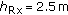as a function of the distance BS-Rx (the histograms will be explained in Section 5). Each marker on the curve of a certain model corresponds with a modulation scheme and its range. BPSK 1/2 corresponds with the highest ranges while 64-QAM will reach the shortest distances. BPSK 1/2 results in ranges up to 0.77 km for model 1, 0.63 km for model 2 and 0.78 km for the model of , terrain C, that is, suburban regions with flat terrain and low tree density, while 64-QAM 3/4 is only possible up to about 0.24 km for model 1, 0.29 km for model 2 and 0.33 km for the model of .

Figure 6 shows a reasonable correspondance between the range estimations of the different path loss models for suburban environments. The deviations are due to differences in environmental model types (one or two parameters), and the used equipment (such as the WiMAX base station, and antennas). When coverage estimations are made, the used path loss model should be carefully selected. How these ranges correspond with the true performance will be analyzed in Section 5.

## 5. Validation

Figure 6 shows the results of the actual performance measurements executed on the used WiMAX system at different locations. The different modulation schemes (left-axis) as a function of the distance are shown. At each location (corresponding with a certain distance from the BS), the used modulation scheme is monitored (see Section 3.2) each second during a period of 12 minutes (in total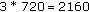samples per location). Thus each modulation scheme (MS) can occur several times at a certain distance.

For each modulation scheme separately (right-axis), the different zones where the modulation scheme occurs are collected and classified into a histogram by splitting the range of the data into equal-sized bins (or zones) of 100 m (starting from 0 m until 1300 m, see Section 3.2). Each histogram in Figure 6 shows the ratio of the number of the samples belonging to each zone to the total number of samples of a modulation scheme. These histograms show thus how the different modulation schemes are distributed as a function of the distance to the BS. This ratio is noted as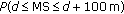withdistance BS-Rx, or the probability that a certain modulation scheme occurs in a zonetofrom the BS. Figure 6 shows, for example, that 64-QAM 3/4 only appears up to a distance of 400 m and reaches a maximum between 200 m and 300 m (with a probability of about 0.65). BPSK occurs from 500 m up to 1300 m, with a maximal probability of 0.3 in the zone between 900 m and 1000 m.

A relative high correlation coefficient of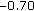between modulation scheme and distance is obtained: the larger the distance, the lower the modulation scheme. However, this correlation is lower than the correlation coefficients of the path loss models, which is about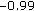(almost perfectly correlated). The lower correlation of the true performance is due to the significant influence of "local factors'' (such as high buildings, small streets), which can largely degrade the performance at the receiver side. Path loss models take these circumstances less into account. Therefore one should be careful when using these path loss models to estimate the range of a particular WiMAX system.

The curve "avg performance meas'' (Figure 6) is calculated by averaging the samples for each modulation scheme. This curve shows the average range corresponding to a certain modulation scheme. BPSK 1/2 (and QPSK 1/2) corresponds again with the highest ranges while 64-QAM will reach the shortest distances. BPSK 1/2 and QPSK 1/2 reach about the same range (for the true performance) due to the limited number of locations that have been investigated (more locations would result in higher ranges for BPSK 1/2 than for QPSK 1/2). Comparing the curve of the average performance with the curves of the link budget calculations of the different path loss models, a reasonable correspondance can be observed for all path loss models. While the average performance of BPSK 1/2 and 64-QAM 3/4 corresponds with ranges 0.97 km and 0.26 km, the link budget calculations for, for example, model 1 correspond with ranges of 0.77 km and 0.24 km, respectively. The differences between link budget calculations and average actual performance may be due to the fact that the performance measurements with the CPEs are performed with directional CPE antennas that always have been oriented in the direction where the RSSI reaches a maximum (see Section 3.2).

This study shows that one has to be careful when performing link budget calculations for suburban environments. These calculations can thus be used to make a rough estimation of the number of required base stations for the deployment of WiMAX in a suburban environment (shown by reasonably agreement with average performance). A more detailed site-survey (such as the method described in Section 3.2) is advised for actual deployment of a WiMAX system.

## 6. Conclusions

In this paper propagation measurements and actual performance tests for fixed wireless systems operating at 3.52 GHz are analyzed and discussed. A statistical path loss model at 3.5 GHz for a base station antenna in a suburban environment is developed and link budget calculations are performed using different models. The calculations are validated using performance measurements of a real WiMAX system. Average performance results based on measurements correspond reasonably well with the ranges calculated with different path loss models. While link budget calculations are almost perfectly correlated with distance, the actual performance measurements are less correlated with the distance. Path loss models can be used to make an estimation of the number of required base stations within a specific environment, but a detailed site-survey is advised for actual deployment of the WiMAX base stations.

## References

1. IEEE Std. 802.16—IEEE standard for local and metropolitan area networks : Part 16: air interface for fixed broadband wireless access systems. 2004.

2. ETSI : ETSI broadband radio access networks (BRAN) HIPERMAN physical (PHY) layer. Standards TS 102 177. 2003.

3. Joseph W, Martens L: Performance evaluation of broadband fixed wireless system based on IEEE 802.16. Proceedings of the IEEE Wireless Communications and Networking Conference (WCNC '06), April 2006, Las Vegas, Nev, USA 2: 978-983.

4. Joseph W, Reynders W, De Bruyne J, Martens L: Influence of channel models and MIMO on the performance of a system based on IEEE 802.16. Proceedings of the IEEE Wireless Communications and Networking Conference (WCNC '07), March 2007, Hong Kong 1826-1830.

5. Abhayawardhana VS, Wassellt IJ, Crosby D, Sellars MP, Brown MG: Comparison of empirical propagation path loss models for fixed wireless access systems. Proceedings of the IEEE Vehicular Technology Conference (VTC '05), May 2005, Stockholm, Sweden 1(1):73-77.

6. Ball CF, Humburg E, Ivanov K, Treml F: Comparison of IEEE 802.16 WiMAX scenarios with fixed and mobile subscribers in tight reuse. European Transactions on Telecommunications 2006, 17(2):203-218. 10.1002/ett.1102

7. Nuaymi L, Noun Z: Simple capacity estimations in WiMAX/802.16 system. Proceedings of the IEEE International Symposium on Personal, Indoor and Mobile Radio Communications (PIMRC '06), September 2006, Helsinki, Finland 1-5.

8. Li J, Haggman S-G: Performance of IEEE 802.16-2004 based system in jamming environment and its improvement with link adaptation. Proceedings of the International Symposium on Personal, Indoor and Mobile Radio Commumications (PIMRC '06), September 2006, Helsinki, Finland 1-5.

9. Ghosh A, Andrews JG, Chen R, Wolter DR: Broadband wireless access with WiMax/802.16: current performance benchmarks, and future potential. IEEE Communications Magazine 2005, 43(2):129-136.

10. Hoymann C, Puttner M, Forkel I: The HIPERMAN standard—a performance analysis. Proceedings of the IST Mobile & Communication Summit, June 2003, Aveiro, Portugal 827-831.

11. Rial AV, Kraus H, Hauck J, Buchholz M: Measurements and analysis of a WiMAX field trial at 3.5 GHz in an urban environment. Proceedings of IEEE International Symposium on Broadband Multimedia Systems and Broadcasting (BMSB '07), March 2007, Orlando, Fla, USA

12. Tsourakis T, Voudouris K: WiMAX network planning and system's performance evaluation. Proceedings of the IEEE Wireless Communications and Networking Conference (WCNC '07), March 2007, Hong Kong 1948-1953.

13. De Bruyne J, Joseph W, Martens L, Olivier C, De Ketelaere W: Field measurement and performance analysis of an 802.16 system in a suburban environment. IEEE Transactions on Wireless Communications 2009, 8(3):1424-1434.

14. ETSI EN 301 021 V1.6.1 : Fixed radio systems; point-to-multipoint equipment; time division multiple access (TDMA); point-to-multipoint digital radio systems in frequency bands in the range 3 GHz to 11 GHz. 2003–2007.

15. Jaybeam : MA431X21. http://www.amphenol-jaybeam.com/datasheet_files/MA431X21.pdf

16. ITU-R Recommendation SM.1708 : Field-strength measurements along a route with geographical coordinate registrations. 2005.

17. Lee WCY: Mobile Communications Design Fundamentals. John Wiley & Sons, New York, NY, USA; 1993.

18. Erceg V, Greenstein LJ, Tjandra S, et al.: An empirically based path loss model for wireless channels in suburban environments. IEEE Journal on Selective Areas in Communication 1999, 17(7):1205-1211. 10.1109/49.778178

19. Saunders SR: Antennas and Propagation for Wireless Communication Systems. John Wiley & Sons, New York, NY, USA; 1999.

20. ITU-R Recommendation P.530-10 : Propagation data and prediction methods required for the design of terrestrial line-of-sight systems.

21. ECC Report 33 : The analysis of the coexistence of FWA cells in the 3.4–3.8 GHz band. 2003.

## Acknowledgments

This work was supported by the IBBT-CIcK project, co-funded by the Interdisciplinary Institute for BroadBand Technology (IBBT), a research institute founded by the Flemish Government in 2004, and the involved companies and institutions. W. Joseph is a Postdoctoral Fellow of the FWO-V (Research Foundation at Flanders).

## Author information

Authors

### Corresponding author

Correspondence to Wout Joseph.

## Rights and permissions

Reprints and Permissions

De Bruyne, J., Joseph, W., Plets, D. et al. Comparison of the Link Budget with Experimental Performance of a WiMAX System. J Wireless Com Network 2009, 247436 (2009). https://doi.org/10.1155/2009/247436

• Revised:

• Accepted:

• Published:

• DOI: https://doi.org/10.1155/2009/247436

### Keywords

• Path Loss
• Modulation Scheme